# Kerala Board Question Paper for SSLC Class 10th Physics 2018 In PDF

## KBPE 10th Standard Physics Exam Question Paper 2018 with Solutions – Free Download

Kerala Board SSLC Class 10 Physics 2018 Question Paper solutions are the perfect resource. It helps the students to prepare most efficiently for the board exams. This resource is used by students to score well in the final exams. If any student is looking to ace the exams, then they are encouraged to practise these solutions regularly. The Class 10 exam question paper sets the foundation for most of the questions that are likely to be repeated in the Physics paper for the final exam. Students can download the PDF formats of the solved or unsolved question paper, as preferred with just a click on the listed links. For the convenience of the students, we have listed the clickable links in this article. Meanwhile, we also provide the questions and answers on the web-page.

All students who are thorough with the complete complex concepts from Physics will find it easier to gain good scores in the final exams. These students will find it easy to understand the question types that are found in the KBPE SSLC Class 10 Physics exam. Students are advised to revise the entire subject before the exam. They can also solve the previous papers of Kerala Board Class 10 Physics, after which, they can refer to the solutions and self-analyse their performance. They can also scale their preparation level for the Class 10 board exams. This will help the students to identify where they have gone wrong while answering the question paper and they can then further avoid these types of errors. These SSLC previous year question papers also give an overview of the question paper design and the marks’ distribution. Students can also gauge the difficulty level of the exams with the help of these question paper solutions.

### Kerala Board Class 10 Physics 2018 Question Paper with Solutions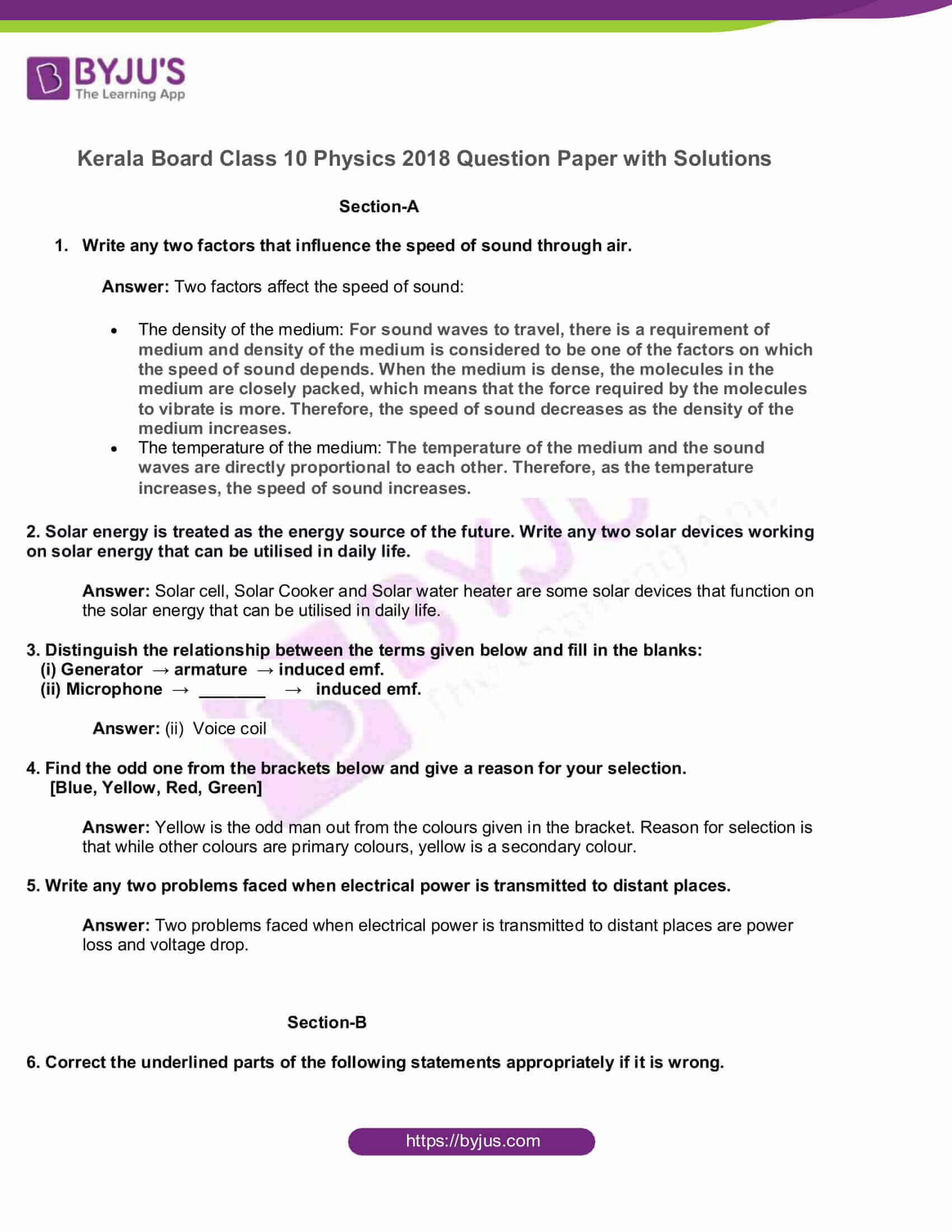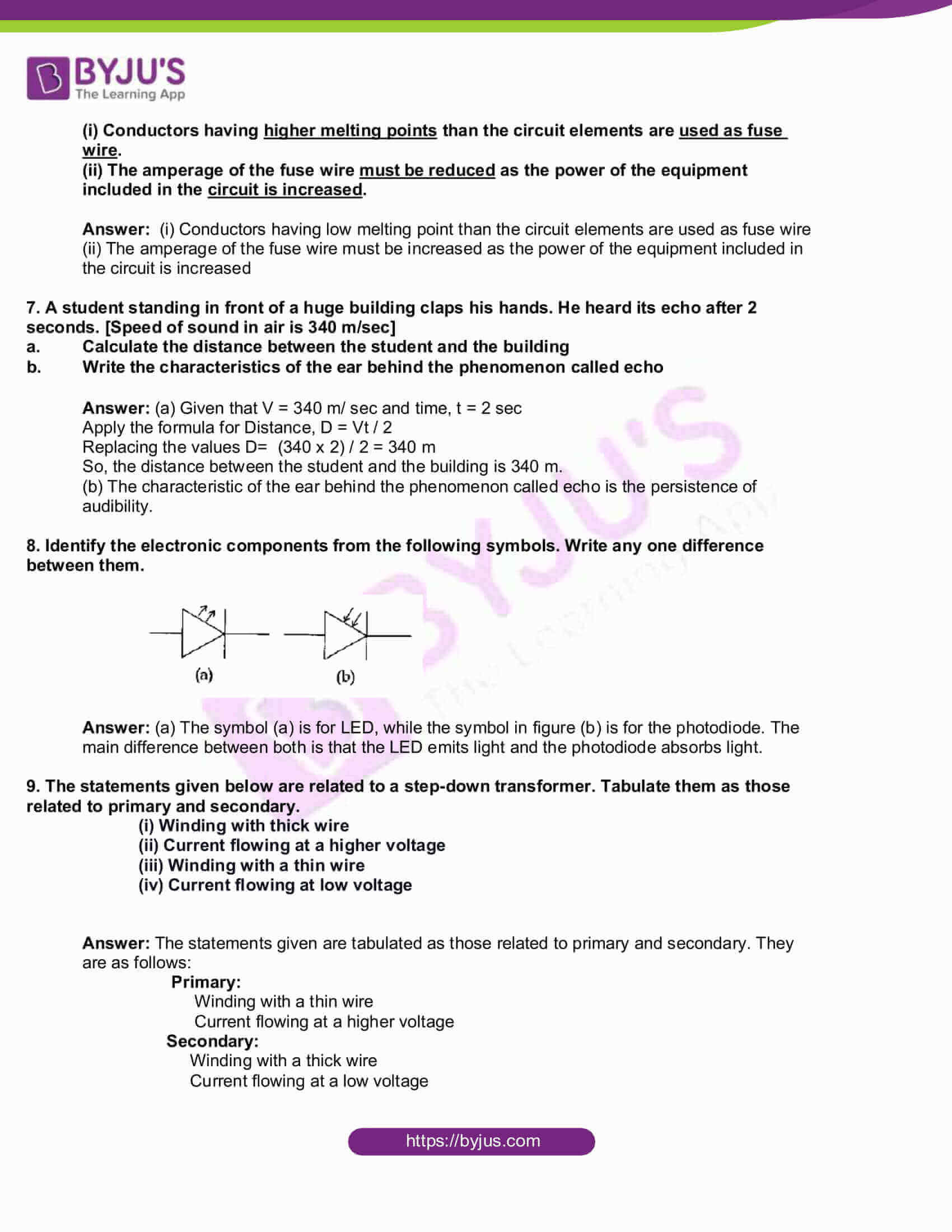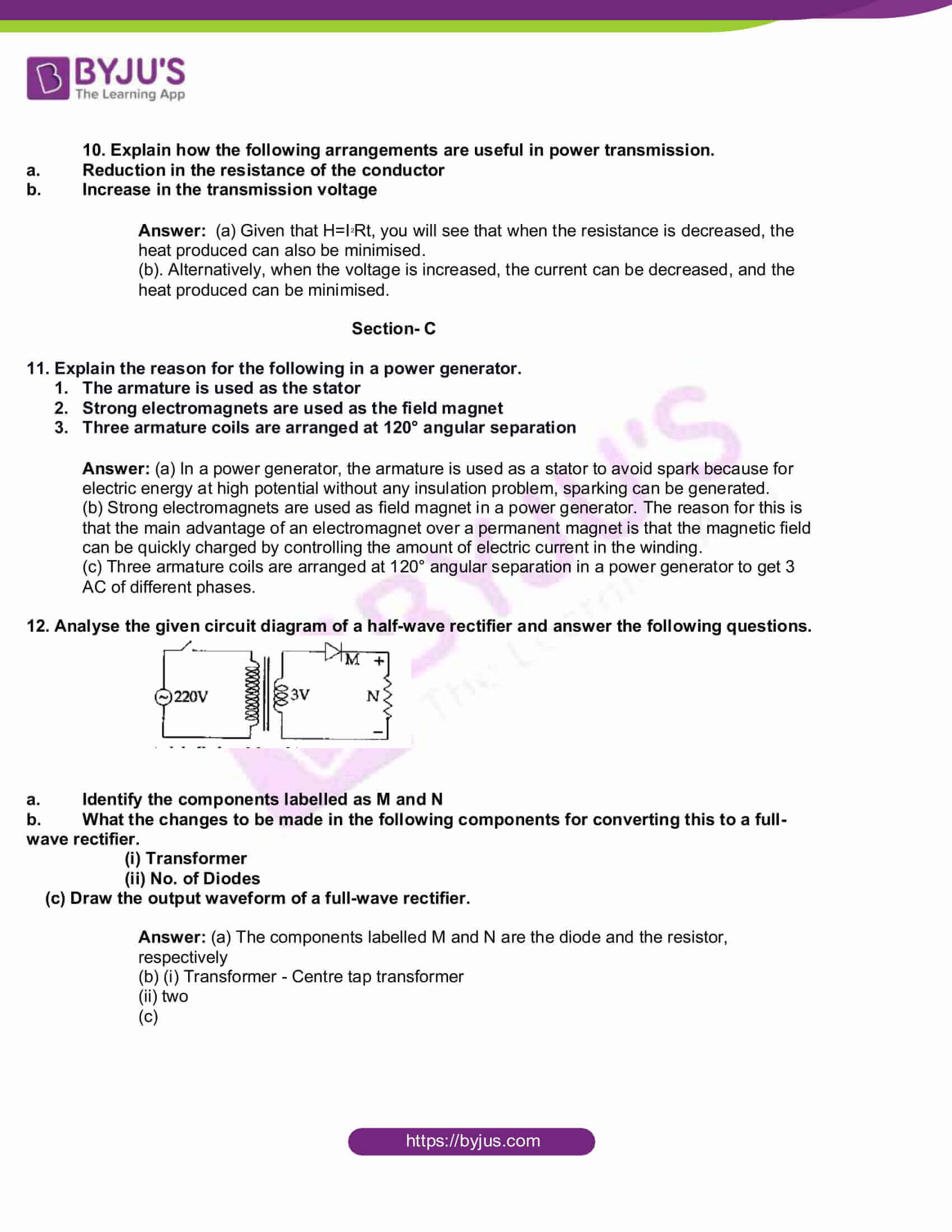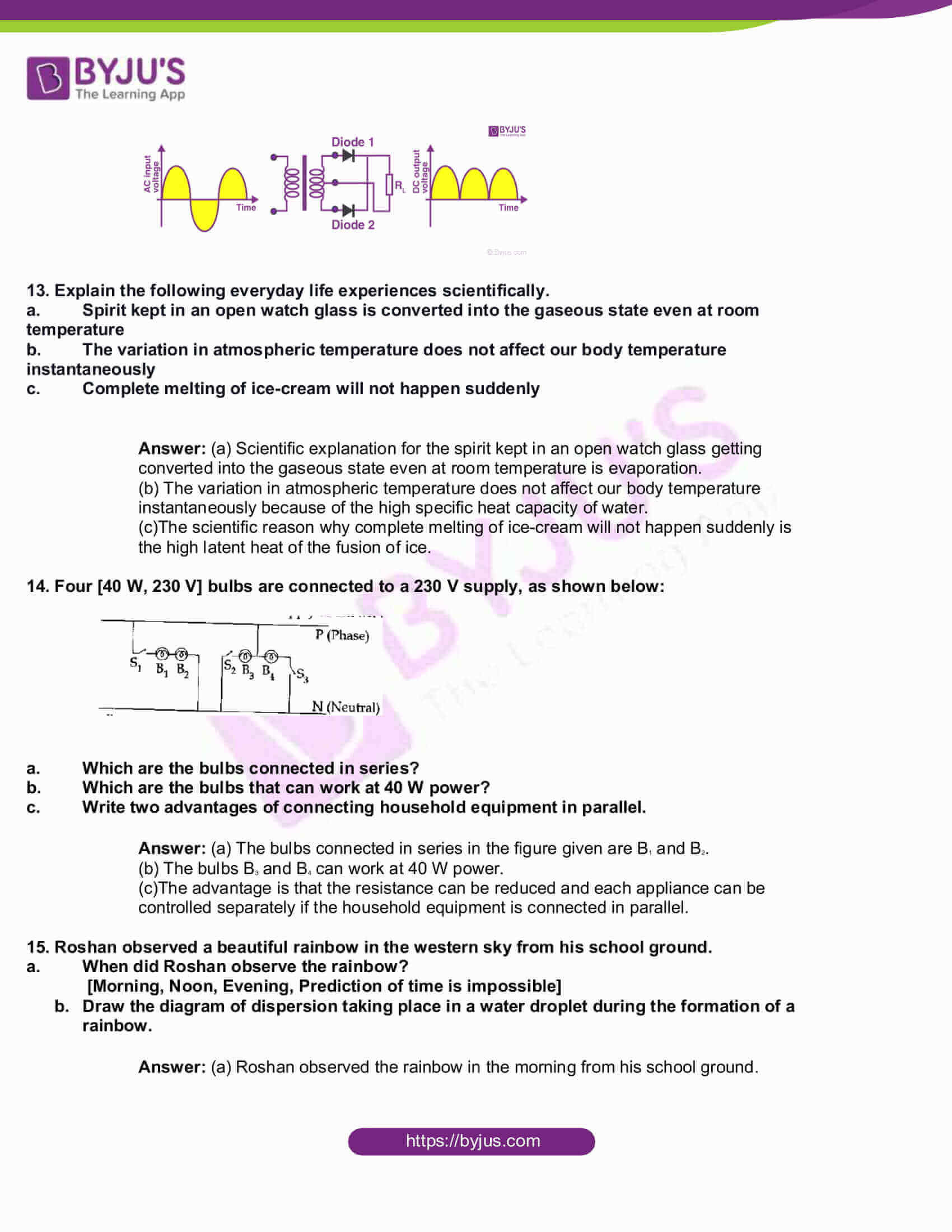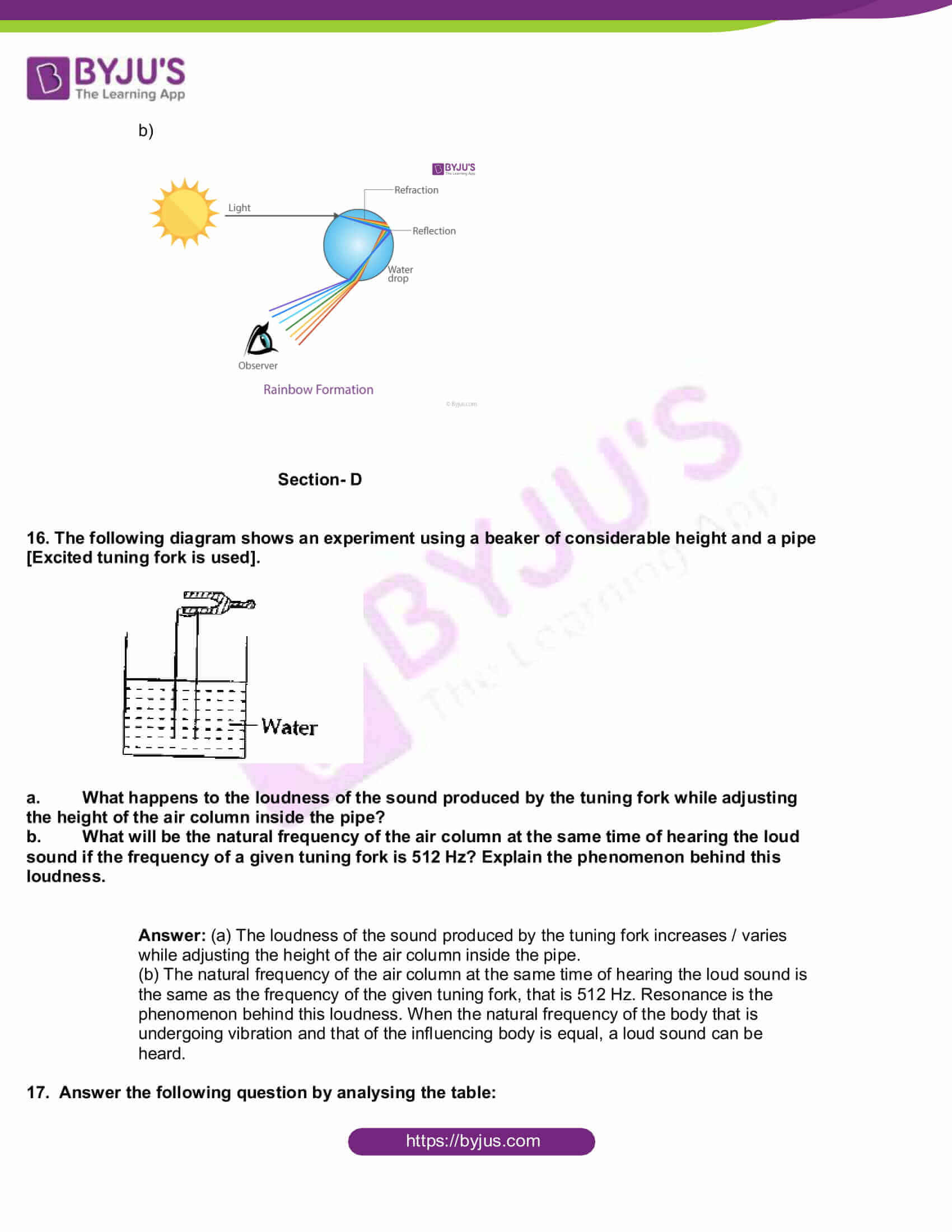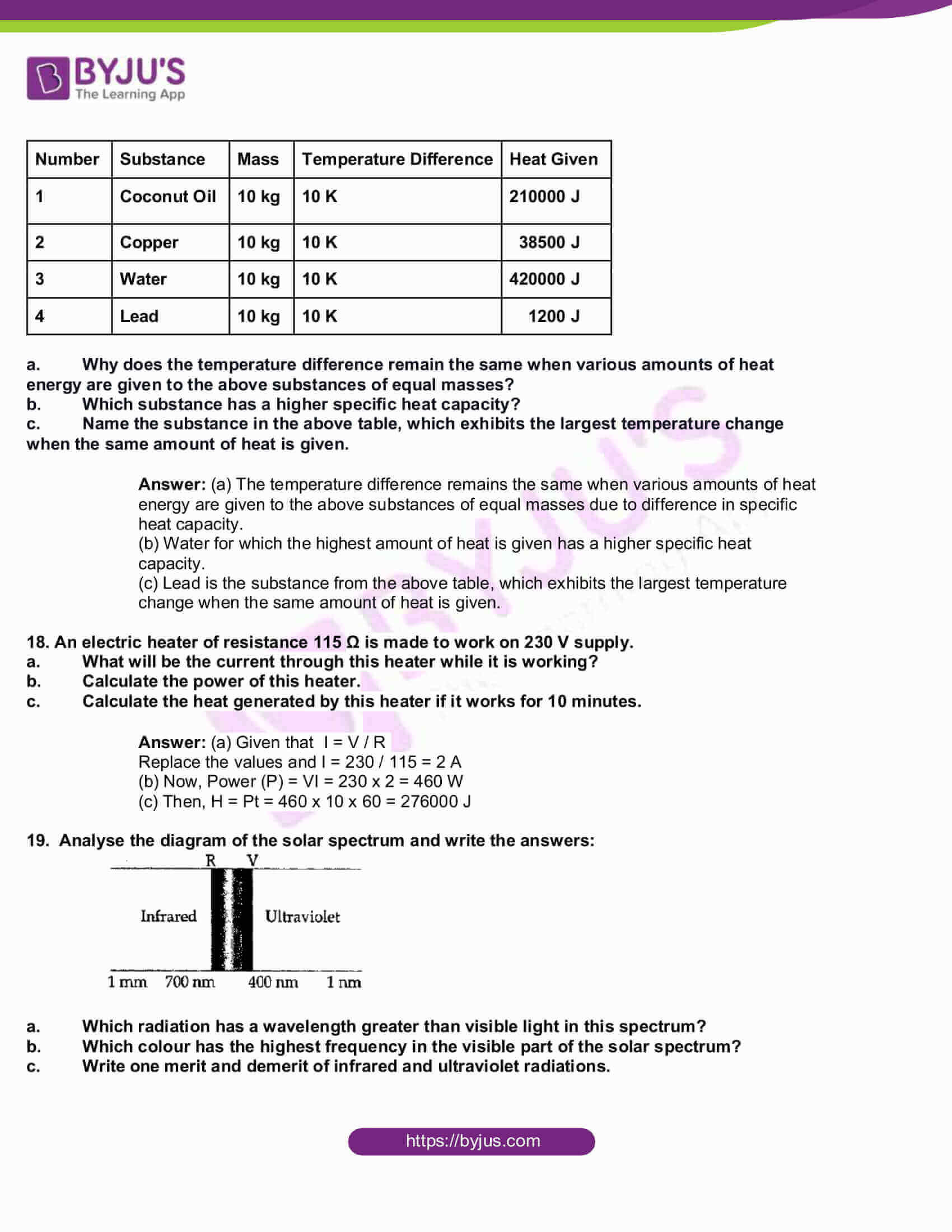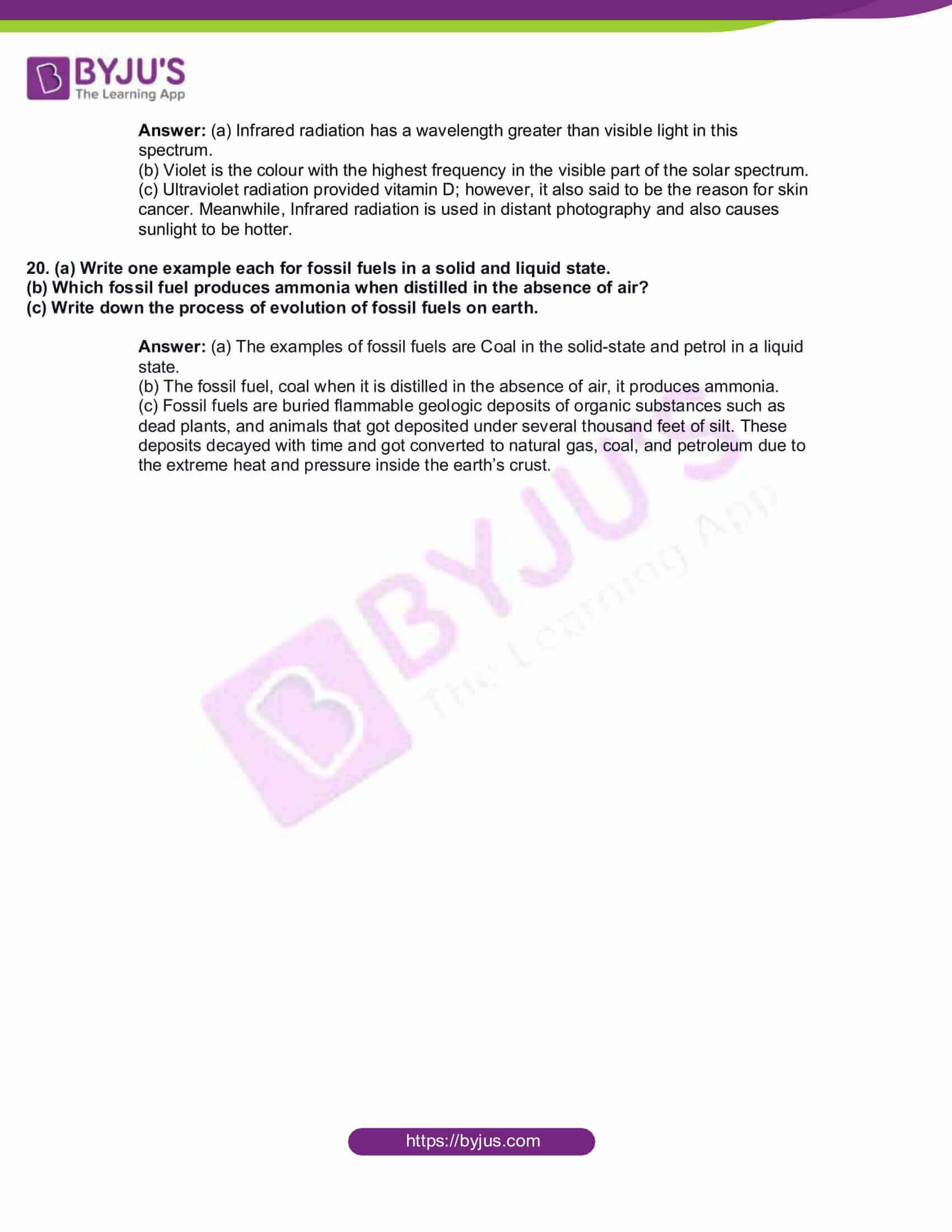Section-A

1. Write any two factors that influence the speed of sound through air.

Answer: Two factors affect the speed of sound:

### The temperature of the medium: The temperature of the medium and the sound waves are directly proportional to each other. Therefore, as the temperature increases, the speed of sound increases.

2. Solar energy is treated as the energy source of the future. Write any two solar devices working on solar energy that can be utilised in daily life.

Answer: Solar cell, Solar Cooker and Solar water heater are some solar devices that function on the solar energy that can be utilised in daily life.

3. Distinguish the relationship between the terms given below and fill in the blanks:

(i) Generator → armature → induced emf.

(ii) Microphone → _______ → induced emf.

4. Find the odd one from the brackets below and give a reason for your selection.

[Blue, Yellow, Red, Green]

Answer: Yellow is the odd man out from the colours given in the bracket. Reason for selection is that while other colours are primary colours, yellow is a secondary colour.

5. Write any two problems faced when electrical power is transmitted to distant places.

Answer: Two problems faced when electrical power is transmitted to distant places are power loss and voltage drop.

Section-B

6. Correct the underlined parts of the following statements appropriately if it is wrong.

(i) Conductors having higher melting points than the circuit elements are used as fuse wire.

(ii) The amperage of the fuse wire must be reduced as the power of the equipment included in the circuit is increased.

Answer: (i) Conductors having low melting point than the circuit elements are used as fuse wire

(ii) The amperage of the fuse wire must be increased as the power of the equipment included in the circuit is increased

7. A student standing in front of a huge building claps his hands. He heard its echo after 2 seconds. [Speed of sound in air is 340 m/sec]

1. Calculate the distance between the student and the building
2. Write the characteristics of the ear behind the phenomenon called echo

Answer: (a) Given that V = 340 m/ sec and time, t = 2 sec

Apply the formula for Distance, D = Vt / 2

Replacing the values D= (340 x 2) / 2 = 340 m

So, the distance between the student and the building is 340 m.

(b) The characteristic of the ear behind the phenomenon called echo is the persistence of audibility.

8. Identify the electronic components from the following symbols. Write any one difference between them.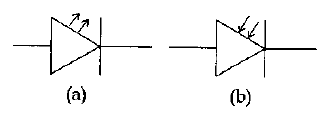Answer: (a) The symbol (a) is for LED, while the symbol in figure (b) is for the photodiode. The main difference between both is that the LED emits light and the photodiode absorbs light.

9. The statements given below are related to a step-down transformer. Tabulate them as those related to primary and secondary.

(i) Winding with thick wire

(ii) Current flowing at a higher voltage

(iii) Winding with a thin wire

(iv) Current flowing at low voltage

Answer: The statements given are tabulated as those related to primary and secondary. They are as follows:

Primary:

Winding with a thin wire

Current flowing at a higher voltage

Secondary:

Winding with a thick wire

Current flowing at a low voltage

10. Explain how the following arrangements are useful in power transmission.

1. Reduction in the resistance of the conductor
2. Increase in the transmission voltage

Answer: (a) Given that H=I2Rt, you will see that when the resistance is decreased, the heat produced can also be minimised.

(b). Alternatively, when the voltage is increased, the current can be decreased, and the heat produced can be minimised.

Section- C

11. Explain the reason for the following in a power generator.

1. The armature is used as the stator
2. Strong electromagnets are used as the field magnet
3. Three armature coils are arranged at 120° angular separation

Answer: (a) In a power generator, the armature is used as a stator to avoid spark because for electric energy at high potential without any insulation problem, sparking can be generated.

(b) Strong electromagnets are used as field magnet in a power generator. The reason for this is that the main advantage of an electromagnet over a permanent magnet is that the magnetic field can be quickly charged by controlling the amount of electric current in the winding.

(c) Three armature coils are arranged at 120° angular separation in a power generator to get 3 AC of different phases.

12. Analyse the given circuit diagram of a half-wave rectifier and answer the following questions.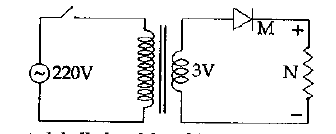1. Identify the components labelled as M and N
2. What the changes to be made in the following components for converting this to a full-wave rectifier.

(i) Transformer

(ii) No. of Diodes

(c) Draw the output waveform of a full-wave rectifier.

Answer: (a) The components labelled M and N are the diode and the resistor, respectively

(b) (i) Transformer – Centre tap transformer

(ii) two

(c)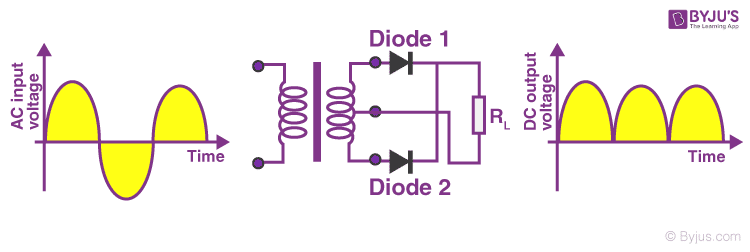13. Explain the following everyday life experiences scientifically.

1. Spirit kept in an open watch glass is converted into the gaseous state even at room temperature
2. The variation in atmospheric temperature does not affect our body temperature instantaneously
3. Complete melting of ice-cream will not happen suddenly

Answer: (a) Scientific explanation for the spirit kept in an open watch glass getting converted into the gaseous state even at room temperature is evaporation.

(b) The variation in atmospheric temperature does not affect our body temperature instantaneously because of the high specific heat capacity of water.

(c)The scientific reason why complete melting of ice-cream will not happen suddenly is the high latent heat of the fusion of ice.

14. Four [40 W, 230 V] bulbs are connected to a 230 V supply, as shown below: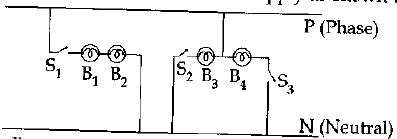1. Which are the bulbs connected in series?
2. Which are the bulbs that can work at 40 W power?
3. Write two advantages of connecting household equipment in parallel.

Answer: (a) The bulbs connected in series in the figure given are B1 and B2.

(b) The bulbs B3 and B4 can work at 40 W power.

(c)The advantage is that the resistance can be reduced and each appliance can be controlled separately if the household equipment is connected in parallel.

15. Roshan observed a beautiful rainbow in the western sky from his school ground.

1. When did Roshan observe the rainbow?

[Morning, Noon, Evening, Prediction of time is impossible]

1. Draw the diagram of dispersion taking place in a water droplet during the formation of a rainbow.

Answer: (a) Roshan observed the rainbow in the morning from his school ground.

b)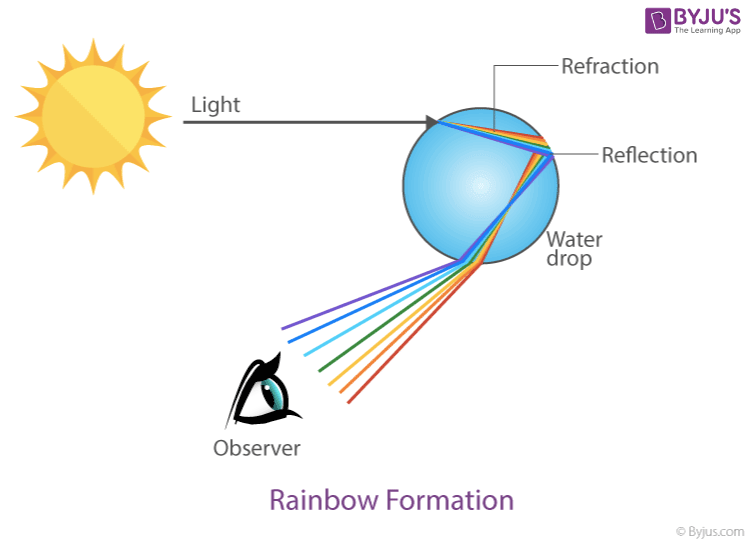Section- D

16. The following diagram shows an experiment using a beaker of considerable height and a pipe [Excited tuning fork is used].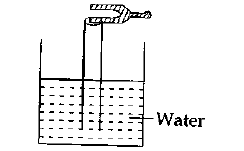1. What happens to the loudness of the sound produced by the tuning fork while adjusting the height of the air column inside the pipe?
2. What will be the natural frequency of the air column at the same time of hearing the loud sound if the frequency of a given tuning fork is 512 Hz? Explain the phenomenon behind this loudness.

Answer: (a) The loudness of the sound produced by the tuning fork increases / varies while adjusting the height of the air column inside the pipe.

(b) The natural frequency of the air column at the same time of hearing the loud sound is the same as the frequency of the given tuning fork, that is 512 Hz. Resonance is the phenomenon behind this loudness. When the natural frequency of the body that is undergoing vibration and that of the influencing body is equal, a loud sound can be heard.

17. Answer the following question by analysing the table:

 Number Substance Mass Temperature Difference Heat Given 1 Coconut Oil 10 kg 10 K 210000 J 2 Copper 10 kg 10 K 38500 J 3 Water 10 kg 10 K 420000 J 4 Lead 10 kg 10 K 1200 J
1. Why does the temperature difference remain the same when various amounts of heat energy are given to the above substances of equal masses?
2. Which substance has a higher specific heat capacity?
3. Name the substance in the above table, which exhibits the largest temperature change when the same amount of heat is given.

Answer: (a) The temperature difference remains the same when various amounts of heat energy are given to the above substances of equal masses due to difference in specific heat capacity.

(b) Water for which the highest amount of heat is given has a higher specific heat capacity.

(c) Lead is the substance from the above table, which exhibits the largest temperature change when the same amount of heat is given.

18. An electric heater of resistance 115 Ω is made to work on 230 V supply.

1. What will be the current through this heater while it is working?
2. Calculate the power of this heater.
3. Calculate the heat generated by this heater if it works for 10 minutes.

Answer: (a) Given that I = V / R

Replace the values and I = 230 / 115 = 2 A

(b) Now, Power (P) = VI = 230 x 2 = 460 W

(c) Then, H = Pt = 460 x 10 x 60 = 276000 J

19. Analyse the diagram of the solar spectrum and write the answers: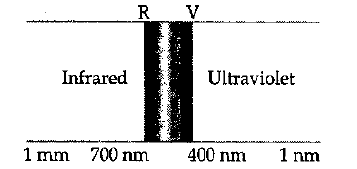1. Which radiation has a wavelength greater than visible light in this spectrum?
2. Which colour has the highest frequency in the visible part of the solar spectrum?
3. Write one merit and demerit of infrared and ultraviolet radiations.

Answer: (a) Infrared radiation has a wavelength greater than visible light in this spectrum.

(b) Violet is the colour with the highest frequency in the visible part of the solar spectrum.

(c) Ultraviolet radiation provided vitamin D; however, it also said to be the reason for skin cancer. Meanwhile, Infrared radiation is used in distant photography and also causes sunlight to be hotter.

20. (a) Write one example each for fossil fuels in a solid and liquid state.

(b) Which fossil fuel produces ammonia when distilled in the absence of air?

(c) Write down the process of evolution of fossil fuels on earth.

Answer: (a) The examples of fossil fuels are Coal in the solid-state and petrol in a liquid state.

(b) The fossil fuel, coal when it is distilled in the absence of air, it produces ammonia.

(c) Fossil fuels are buried flammable geologic deposits of organic substances such as dead plants, and animals that got deposited under several thousand feet of silt. These deposits decayed with time and got converted to natural gas, coal, and petroleum due to the extreme heat and pressure inside the earth’s crust.